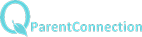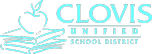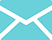The major themes behind Chapter 10 are...

1.    Dependent and Independent Samples

a.    Understanding the difference between

independent and dependent samples.

i.    Dependent is a comparison between two

values.  Ordered Pairs.

ii.   Independent is when two samples could

stand alone.  Samples Size

doesn’t have to be the same.

2.    Inference Concerning the Mean Difference.

Dependent Samples

a.    Must choose the correct Alternative

Hypothesis.

i.    Subtraction between the ordered pairs is

important.

b.    Use of t-distribution

i.    Same as Last Chapter.

c.    Hypothesis Testing Concerning Mean

Difference (Dependent) and

Confidence Interval

i.    State Null and Alternative Hypothesis/

State Estimation

ii.    Verify Conditions

1)    Check SRS

2)    Check Normality (Box and

Whiskers, Probability Plot, etc.)

3)    Check Sample Size.  (Number of

ordered pairs)

iii.    Calculations.

iv.    Explanation of calculation.

3.    Inferences Concerning the Mean Difference.

Independent Samples

a.    Must choose the correct Alternative

Hypothesis

b.    Hypothesis Testing Concerning Concerning

Mean Difference and

Confidence Interval

i.    State Null and Alternative Hypothesis/

State Estimation

ii.    Verify Conditions

1)    Check SRS

2)    Check Normality (Box and

Whiskers, Probability Plot, etc.)

3)    Check Sample Size.  (Use smaller

Sample Size for df)

iii.    Calculations

iv.    Explanation of calculation.

4.    Inferences Concerning the Proportion Difference.

a.    Must choose the correct Alternative

Hypothesis

b.    Hypothesis Testing Concerning Concerning

Proportion Differnce and

Confidence Interval

i.    State Null and Alternative Hypothesis/

State Estimation

ii.    Verify Conditions

1)    Check SRS

2)    Check np and nq (All 4 must satisfy

the condition)

3)    Check Sample Size.  Sample Size

< 1/10 of Population

iii.    Calculations (Watch out on

Testing...different Standard Error)

iv.    Explanation of calculation.

Statistics

Syllabus

Statistics and ProbabilityBuchanan High School     |     1560 N. Minnewawa Ave.    |     Clovis, CA     |     93619     |     (559) 327-3000